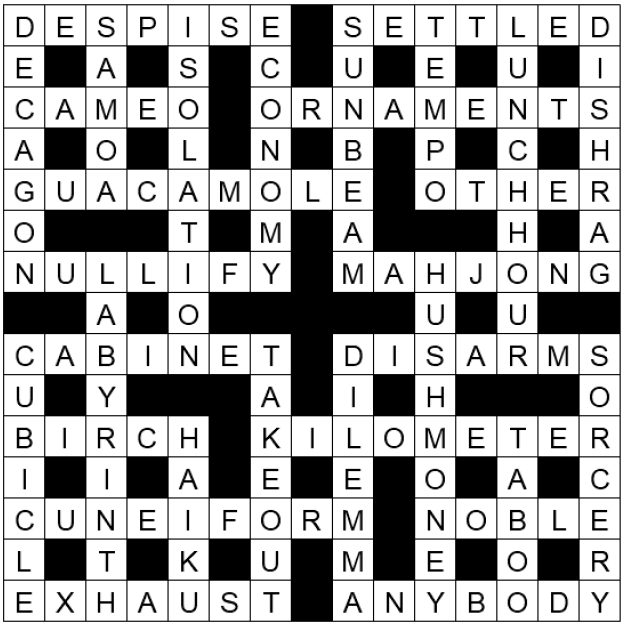This is a variety crossword

## Context

This puzzle was one of the puzzles we wrote to include in our wedding book.

## Hints

The bottom-left corner is a great place to start here. There are two 5s (one across, one down), a 126, and a 124, and they are forced to be specific pairs of numbers. The Rhode Island specific clue you may want to google, the type of farm is not really a farm, and the consume clue should be thought of in terms of resources. From here you can either start working on potential crossings (how many 5-letter words end in a U?), or look for clues with numbers that are really high or really low.Across
5+13 = DESPISE
6+15 = SETTLED
37+45 = CAMEO
24+30 = ORNAMENTS
34+35 = GUACAMOLE
38+47 = OTHER
32+40 = NULLIFY
8+59 = MAJHONG
1+4 = CABINET
51+60 = DISARMS
52+56 = BIRCH
17+46 = KILOMETER
55+58 = CUNEIFORM
16+25 = NOBLE
62+64 = EXHAUST
19+31 = ANYBODY
Down
7+11 = DECAGON
2+3 = CUBICLE
49+54 = SAMOA
10+14 = LABYRINTH
18+27 = ISOLATION
21+39 = HAIKU
43+50 = ECONOMY
61+63 = TAKEOUT
9+12 = SUNBEAM
53+58 = DILEMMA
28+33 = TEMPO
20+48 = HUSH MONEY
26+36 = LUNCH HOUR
22+44 = TABOO
29+41 = DISHRAG
23+42 = SORCERY